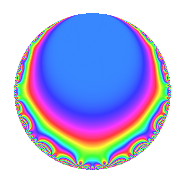# Properties

 Label 119.1.d.aLevel 119 Weight 1 Character orbit 119.d Self dual Yes Analytic conductor 0.059 Analytic rank 0 Dimension 2 Projective image $$D_{5}$$ CM disc. -119 Inner twists 2

# Related objects

## Newspace parameters

 Level: $$N$$ = $$119 = 7 \cdot 17$$ Weight: $$k$$ = $$1$$ Character orbit: $$[\chi]$$ = 119.d (of order $$2$$ and degree $$1$$)

## Newform invariants

 Self dual: Yes Analytic conductor: $$0.0593887365033$$ Analytic rank: $$0$$ Dimension: $$2$$ Coefficient field: $$\Q(\sqrt{5})$$ Coefficient ring: $$\Z[a_1, a_2]$$ Coefficient ring index: $$1$$ Projective image $$D_{5}$$ Projective field Galois closure of 5.1.14161.1 Artin image size $$10$$ Artin image $D_5$ Artin field Galois closure of 5.1.14161.1

## $q$-expansion

Coefficients of the $$q$$-expansion are expressed in terms of $$\beta = \frac{1}{2}(1 + \sqrt{5})$$. We also show the integral $$q$$-expansion of the trace form.

 $$f(q)$$ $$=$$ $$q$$ $$+ ( -1 + \beta ) q^{2}$$ $$+ ( -1 + \beta ) q^{3}$$ $$+ ( 1 - \beta ) q^{4}$$ $$-\beta q^{5}$$ $$+ ( 2 - \beta ) q^{6}$$ $$+ q^{7}$$ $$- q^{8}$$ $$+ ( 1 - \beta ) q^{9}$$ $$+O(q^{10})$$ $$q$$ $$+ ( -1 + \beta ) q^{2}$$ $$+ ( -1 + \beta ) q^{3}$$ $$+ ( 1 - \beta ) q^{4}$$ $$-\beta q^{5}$$ $$+ ( 2 - \beta ) q^{6}$$ $$+ q^{7}$$ $$- q^{8}$$ $$+ ( 1 - \beta ) q^{9}$$ $$- q^{10}$$ $$+ ( -2 + \beta ) q^{12}$$ $$+ ( -1 + \beta ) q^{14}$$ $$- q^{15}$$ $$+ q^{17}$$ $$+ ( -2 + \beta ) q^{18}$$ $$+ q^{20}$$ $$+ ( -1 + \beta ) q^{21}$$ $$+ ( 1 - \beta ) q^{24}$$ $$+ \beta q^{25}$$ $$- q^{27}$$ $$+ ( 1 - \beta ) q^{28}$$ $$+ ( 1 - \beta ) q^{30}$$ $$-\beta q^{31}$$ $$+ q^{32}$$ $$+ ( -1 + \beta ) q^{34}$$ $$-\beta q^{35}$$ $$+ ( 2 - \beta ) q^{36}$$ $$+ \beta q^{40}$$ $$+ ( -1 + \beta ) q^{41}$$ $$+ ( 2 - \beta ) q^{42}$$ $$+ ( -1 + \beta ) q^{43}$$ $$+ q^{45}$$ $$+ q^{49}$$ $$+ q^{50}$$ $$+ ( -1 + \beta ) q^{51}$$ $$-\beta q^{53}$$ $$+ ( 1 - \beta ) q^{54}$$ $$- q^{56}$$ $$+ ( -1 + \beta ) q^{60}$$ $$+ ( -1 + \beta ) q^{61}$$ $$- q^{62}$$ $$+ ( 1 - \beta ) q^{63}$$ $$+ ( -1 + \beta ) q^{64}$$ $$-\beta q^{67}$$ $$+ ( 1 - \beta ) q^{68}$$ $$- q^{70}$$ $$+ ( -1 + \beta ) q^{72}$$ $$+ ( -1 + \beta ) q^{73}$$ $$+ q^{75}$$ $$+ ( 2 - \beta ) q^{82}$$ $$+ ( -2 + \beta ) q^{84}$$ $$-\beta q^{85}$$ $$+ ( 2 - \beta ) q^{86}$$ $$+ ( -1 + \beta ) q^{90}$$ $$- q^{93}$$ $$+ ( -1 + \beta ) q^{96}$$ $$-\beta q^{97}$$ $$+ ( -1 + \beta ) q^{98}$$ $$+O(q^{100})$$ $$\operatorname{Tr}(f)(q)$$ $$=$$ $$2q$$ $$\mathstrut -\mathstrut q^{2}$$ $$\mathstrut -\mathstrut q^{3}$$ $$\mathstrut +\mathstrut q^{4}$$ $$\mathstrut -\mathstrut q^{5}$$ $$\mathstrut +\mathstrut 3q^{6}$$ $$\mathstrut +\mathstrut 2q^{7}$$ $$\mathstrut -\mathstrut 2q^{8}$$ $$\mathstrut +\mathstrut q^{9}$$ $$\mathstrut +\mathstrut O(q^{10})$$ $$2q$$ $$\mathstrut -\mathstrut q^{2}$$ $$\mathstrut -\mathstrut q^{3}$$ $$\mathstrut +\mathstrut q^{4}$$ $$\mathstrut -\mathstrut q^{5}$$ $$\mathstrut +\mathstrut 3q^{6}$$ $$\mathstrut +\mathstrut 2q^{7}$$ $$\mathstrut -\mathstrut 2q^{8}$$ $$\mathstrut +\mathstrut q^{9}$$ $$\mathstrut -\mathstrut 2q^{10}$$ $$\mathstrut -\mathstrut 3q^{12}$$ $$\mathstrut -\mathstrut q^{14}$$ $$\mathstrut -\mathstrut 2q^{15}$$ $$\mathstrut +\mathstrut 2q^{17}$$ $$\mathstrut -\mathstrut 3q^{18}$$ $$\mathstrut +\mathstrut 2q^{20}$$ $$\mathstrut -\mathstrut q^{21}$$ $$\mathstrut +\mathstrut q^{24}$$ $$\mathstrut +\mathstrut q^{25}$$ $$\mathstrut -\mathstrut 2q^{27}$$ $$\mathstrut +\mathstrut q^{28}$$ $$\mathstrut +\mathstrut q^{30}$$ $$\mathstrut -\mathstrut q^{31}$$ $$\mathstrut +\mathstrut 2q^{32}$$ $$\mathstrut -\mathstrut q^{34}$$ $$\mathstrut -\mathstrut q^{35}$$ $$\mathstrut +\mathstrut 3q^{36}$$ $$\mathstrut +\mathstrut q^{40}$$ $$\mathstrut -\mathstrut q^{41}$$ $$\mathstrut +\mathstrut 3q^{42}$$ $$\mathstrut -\mathstrut q^{43}$$ $$\mathstrut +\mathstrut 2q^{45}$$ $$\mathstrut +\mathstrut 2q^{49}$$ $$\mathstrut +\mathstrut 2q^{50}$$ $$\mathstrut -\mathstrut q^{51}$$ $$\mathstrut -\mathstrut q^{53}$$ $$\mathstrut +\mathstrut q^{54}$$ $$\mathstrut -\mathstrut 2q^{56}$$ $$\mathstrut -\mathstrut q^{60}$$ $$\mathstrut -\mathstrut q^{61}$$ $$\mathstrut -\mathstrut 2q^{62}$$ $$\mathstrut +\mathstrut q^{63}$$ $$\mathstrut -\mathstrut q^{64}$$ $$\mathstrut -\mathstrut q^{67}$$ $$\mathstrut +\mathstrut q^{68}$$ $$\mathstrut -\mathstrut 2q^{70}$$ $$\mathstrut -\mathstrut q^{72}$$ $$\mathstrut -\mathstrut q^{73}$$ $$\mathstrut +\mathstrut 2q^{75}$$ $$\mathstrut +\mathstrut 3q^{82}$$ $$\mathstrut -\mathstrut 3q^{84}$$ $$\mathstrut -\mathstrut q^{85}$$ $$\mathstrut +\mathstrut 3q^{86}$$ $$\mathstrut -\mathstrut q^{90}$$ $$\mathstrut -\mathstrut 2q^{93}$$ $$\mathstrut -\mathstrut q^{96}$$ $$\mathstrut -\mathstrut q^{97}$$ $$\mathstrut -\mathstrut q^{98}$$ $$\mathstrut +\mathstrut O(q^{100})$$

## Character Values

We give the values of $$\chi$$ on generators for $$\left(\mathbb{Z}/119\mathbb{Z}\right)^\times$$.

 $$n$$ $$52$$ $$71$$ $$\chi(n)$$ $$-1$$ $$-1$$

## Embeddings

For each embedding $$\iota_m$$ of the coefficient field, the values $$\iota_m(a_n)$$ are shown below.

For more information on an embedded modular form you can click on its label.

Label $$\iota_m(\nu)$$ $$a_{2}$$ $$a_{3}$$ $$a_{4}$$ $$a_{5}$$ $$a_{6}$$ $$a_{7}$$ $$a_{8}$$ $$a_{9}$$ $$a_{10}$$
118.1
 −0.618034 1.61803
−1.61803 −1.61803 1.61803 0.618034 2.61803 1.00000 −1.00000 1.61803 −1.00000
118.2 0.618034 0.618034 −0.618034 −1.61803 0.381966 1.00000 −1.00000 −0.618034 −1.00000
 $$n$$: e.g. 2-40 or 990-1000 Significant digits: Format: Complex embeddings Normalized embeddings Satake parameters Satake angles

## Inner twists

Char. orbit Parity Mult. Self Twist Proved
1.a Even 1 trivial yes
119.d Odd 1 CM by $$\Q(\sqrt{-119})$$ yes

## Hecke kernels

This newform can be constructed as the kernel of the linear operator $$T_{3}^{2}$$ $$\mathstrut +\mathstrut T_{3}$$ $$\mathstrut -\mathstrut 1$$ acting on $$S_{1}^{\mathrm{new}}(119, [\chi])$$.